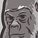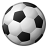# New to Qlik Sense

If you’re new to Qlik Sense, start with this Discussion Board and get up-to-speed quickly.

Announcements
QlikWorld online is next week! REGISTER NOW
cancel
Showing results for
Did you mean:Contributor III

## If (Field A > Field B ), Then Sum(Calculation

Hello!

Search the forum but could not find any awnsers to my question:

I'm trying to make a #kpi like this:

IF(Field A > Field B, Sum((Field C - Field D) * Field E))

Correction: IF((Field A > Field B, Sum((Field A - Field B)*Field C))

But i can't seem to get to right answer, can anybody help?

1 Solution

Accepted SolutionsMVP

If all three fields are numeric, your expression should work with a sum() outside 'if' in KPI object, i.e.

Sum( If (A > B,  ((A - B) * C))  )

If this is not working in KPI object, check if field C is being treated as text or not and correct that accordingly. Otherwise, try to share a sample app.

8 RepliesPartner

Hi there

I'm guessing you have many values in Field A and Field B? If so I think you're going to do an aggregation on those fields to use it in a KPI object. For example IF(Sum(Field A) > Sum(Field B), Sum((Field C - Field D) * Field E)).

Hope this helps.

Regards,

MauritzSpecialist

Hi!

If the another answer doesn't works, you could try

sum (if([Field A]>[Field B], ([Field C]-[Field D])*[Field E]))Specialist III

Have you tried

sum(IF(Field A > Field B,

(Field C - Field D) * Field E

))Contributor III
Author

This doesn't work, Field A & Field B are numeric field (values range from 0 to 50)Partner

Can you perhaps paste a short extract of your data with the expected output?

Regards,

MauritzContributor III
Author
 A B C 5 3 10,54 10 6 14,47 2 1 25,64 3 2 12,31 2 2 8,64 6 8 4,89 2 3 1,58 2 1 33,47 1 1 89,17

I wrote my formula wrong in my post earlier, here is an example piece.

If (A > B,  ((A minus B) * C))

And then i want a total sum of all rows where A is bigger then B in a KPI.MVP

If all three fields are numeric, your expression should work with a sum() outside 'if' in KPI object, i.e.

Sum( If (A > B,  ((A - B) * C))  )

If this is not working in KPI object, check if field C is being treated as text or not and correct that accordingly. Otherwise, try to share a sample app.Contributor III
Author

Got the KPI working, thx a lot everbody for the quick respones!